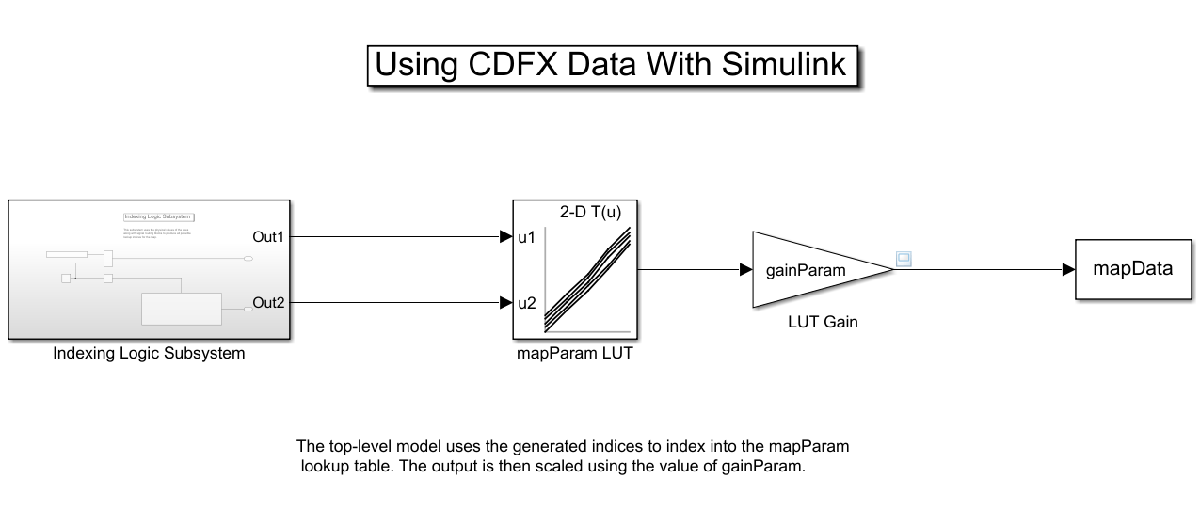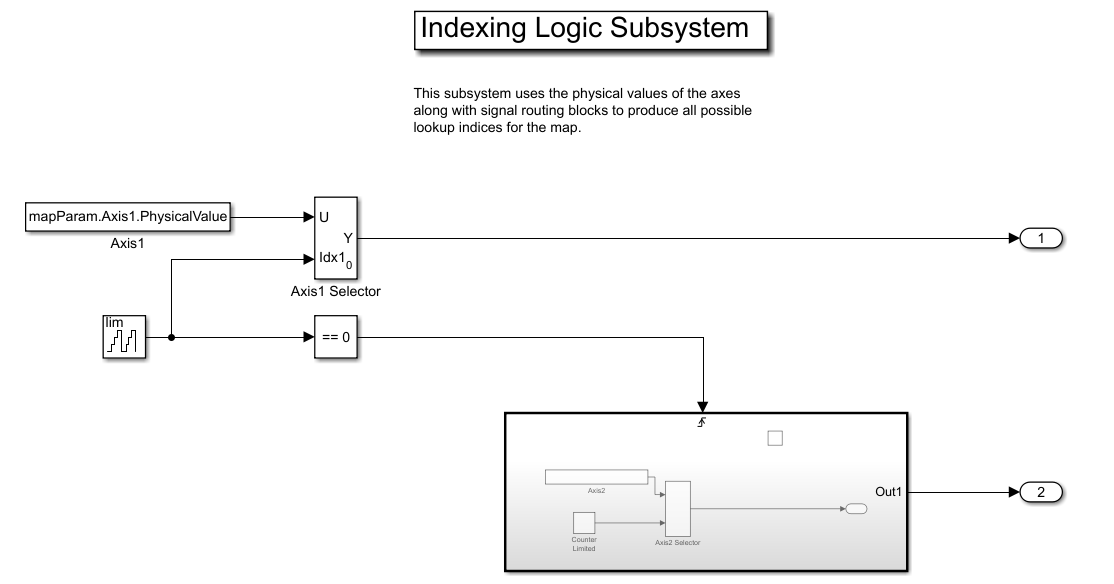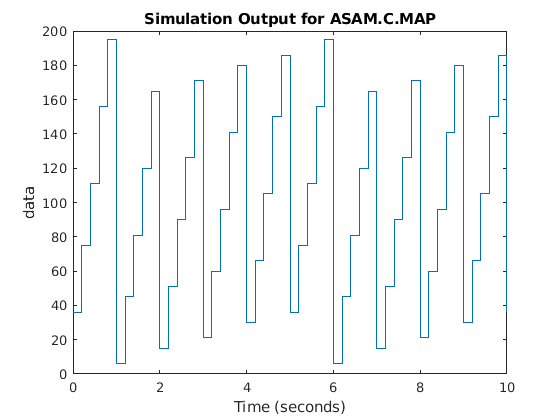# Using ASAM CDFX Data With Simulink Data Dictionary

This example shows how to store calibration data from an ASAM CDFX file in a data dictionary and use these values as parameters to a Simulink model.

### Import Data

Import the calibration data using the `cdfx` function.

`cdfxObj = cdfx("CDFXExampleFile.cdfx")`
```cdfxObj = CDFX with properties: Name: "CDFXExampleFile.cdfx" Path: "/mathworks/home/rollinb/Documents/MATLAB/Examples/vnt-ex73237310-20190405222527/CDFXExampleFile.cdfx" Version: "CDF20" ```

### Create and Populate Data Dictionary with Calibration Data

Use `getValue` to extract the desired parameters into the MATLAB workspace.

`dictName = "CDFXExampleDD.sldd"`
```dictName = "CDFXExampleDD.sldd" ```
```% Check if dictionary is already in the working folder. if isfile(dictName) % If data dictionary exists, open it. dDict = Simulink.data.dictionary.open(dictName) else % If dictionary does not exist, create it and populate with CDFX data. dDict = Simulink.data.dictionary.create(dictName) ddSection = getSection(dDict, "Design Data") addEntry(ddSection, "gainParam", getValue(cdfxObj, "ASAM.C.SCALAR.GAIN")) addEntry(ddSection, "mapParam", getValue(cdfxObj, "ASAM.C.MAP")) end```
```dDict = Dictionary with properties: DataSources: {0×1 cell} HasAccessToBaseWorkspace: 0 EnableAccessToBaseWorkspace: 0 HasUnsavedChanges: 0 NumberOfEntries: 2 ```
```% Display contents of the data dictionary. listEntry(dDict)```
``` Section Name Status DataSource LastModified LastModifiedBy Class Design Data gainParam CDFXExampleDD.sldd 2019-04-05 22:33 rollinb double Design Data mapParam CDFXExampleDD.sldd 2019-04-05 22:33 rollinb struct ```

Open the Simulink model, then use set param to link the existing data dictionary to your model. This will allow the model to access the values defined within the dictionary.

```open_system("CDFXSLDDModel.slx"); cdfxMdl = gcs```
```cdfxMdl = 'CDFXSLDDModel' ```
`set_param(gcs, 'DataDictionary', dictName)`

We can now close the connection to the data dictionary.

`close(dDict)`

### Lookup-Gain ModelThis model contains:

• 2-D Lookup Table block to represent the `ASAM.C.MAP` parameter from the CDFX file. The "Table data" field represents the physical value of the instance, and the "Breakpoint" fields represent the physical values of the axes.

• Gain block to represent the `ASAM.C.SCALAR.GAIN` parameter from the CDFX file.

• To Workspace block to log the simulation data.

### Indexing Logic Subsystem

The Indexing Logic subsystem uses the physical values of the axes of the `ASAM.C.MAP` parameter, along with signal routing blocks and a triggered subsystem, to produce all valid combinations of lookup indices. This configuration can be useful if you need to test across the full range of possible input values of a calibration parameter.### Log Output Data in MATLAB

The output of the simulation is sent to MATLAB by the To Workspace block, where it is stored as a timeseries object called `mapData`. This data can now be inspected and visualized in the MATLAB workspace.

```sim(cdfxMdl); plot(mapData) title("Simulation Output for ASAM.C.MAP")```% Copyright 2018 The MathWorks, Inc.

## SupportGet trial now